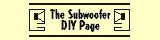Port Length The port length required to tune a volume of air to a specific frequency can be calculated by using the following equation:    Lv = (23562.5*Dv^2*Np/(Fb^2*Vb))-(k*Dv) where,     Dv = port diameter (cm)     Fb = tuning frequency (Hz)     Vb = net volume (litres)     Lv = length of each port (cm)     Np = number of ports      k = end correction (normally 0.732)The value for k, the end correction, can be fine-tuned by using the following values to derive the appropriate end correction figure for each end of the port, then adding them together     Flanged End: 0.425        Free End: 0.307    e.g. if both ends were flanged,            k = 0.425 + 0.425 = 0.850         if one flanged, one free,             k = 0.425 + 0.307 = 0.732                  if both ends were free,            k = 0.307 + 0.307 = 0.614     Normally, k=0.732 is assumed In practice, it's best to use ports that are slightly longer than predicted by the above equations, then adjust their length until the correct tuning is achieved. It is much easier to shorten a port than to lengthen it! Minimum Port Diameter To calculate the minimum diameter of the port required to prevent port noises, you will also need to know the following:     Xmax = maximum linear displacement (mm)     Dia = Effective diameter of driver (cm)     Np = number of ports Calculate the minimum port diameter from the following equations:     Sd = pi*(Dia/100)^2/4     Vd = Sd*Xmax/1000     Dmin = 100*(20.3*(Vd^2/Fb)^0.25)/Np^.5 where,    Dmin = minimum port diameter (cm) Note: You CAN use ports that have a smaller diameter than that given by the equation above, especially if the ports are flared at both ends. However, at higher volumes, you may notice some port noise caused by the air rushing through the ports. Slot Ports If you wish to use a slot port, first determine the diameter of a round port that has the same cross-sectional area as the slot. The following equation can be used to do this:    Dv' = 2*((W*H)/pi)^0.5where,  Dv' =  diameter of equivalent round port  W = width of slot   H = height of slot Once you've calculated Dv', you can use it in the equation for Lv above to determine the required length of the slot port to tune the enclosure to the required frequency (Fb).